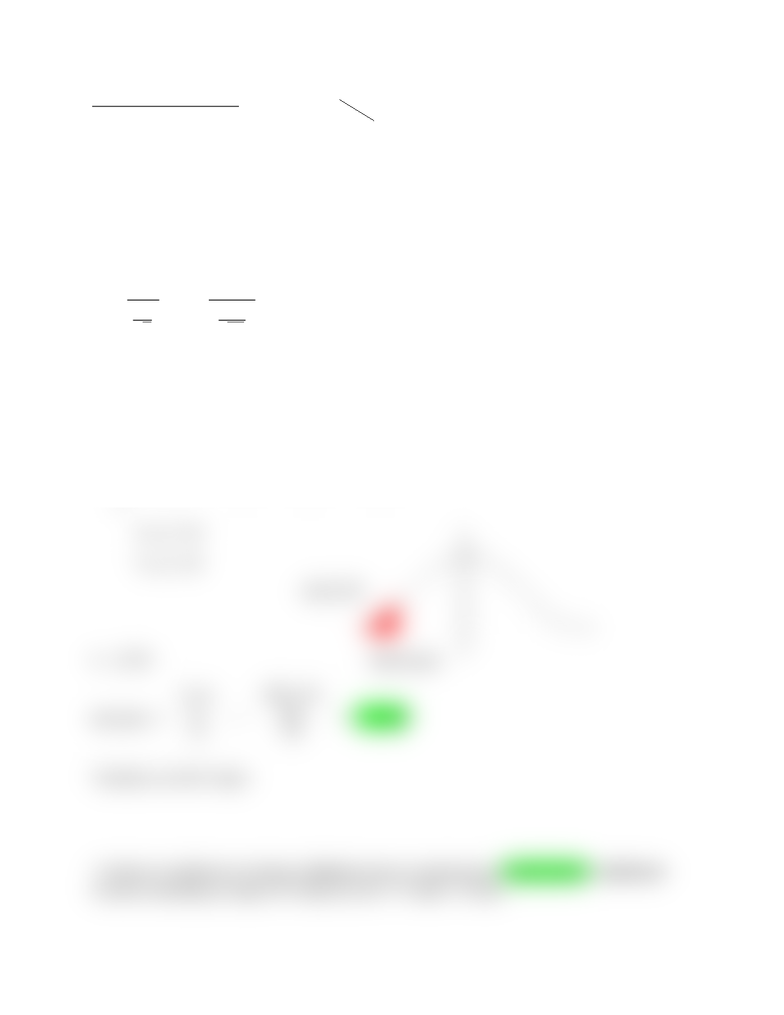Class Notes (1,100,000)
CA (650,000)
Brock U (10,000)
MATH (600)
MATH 1P98 (100)
Lecture

# MATH 1P98 Lecture Notes - Test Statistic

Department
Mathematics and Statistics
Course Code
MATH 1P98
Professor
Dot Miners

This preview shows half of the first page. to view the full 2 pages of the document.Critical Region Method:
Ex: µ = 41 ; x = 47 ; σ = 35 ; α = 0.05 ; n = 40̄
H0: µ = 41
H1: µ > 41
P(z < ?) = 0.05
z0 = 1.645 1 – 0.05 = 0.95
z =
̄
xµ
σ
n
=
4741
35
40
= 1.08  (test statistic)
Now compare test stat to critical value
Therefore, do NOT reject
Ex: µ = 19 ; x = 18.5 ; s = 3.2 ; α = 0.05 ; n = 51̄
H0: µ = 19
H1: µ < 19
t0 = -1.676
test stat: t =
̄
xµ
s
n
=
18.519
3.2
51
= -1.116
Therefore, do NOT reject
**Critical vs traditional z/t testing; Critical involves comparing two critical values, traditional
involves evaluating a range of P-values (0.125 < P-value < 0.250).
area 0.05